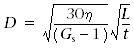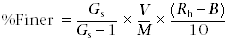IODP Proceedings    Volume contents     Searchdoi:10.2204/iodp.proc.308.205.2008

## Principles of hydrometer analysis

The physical principles of sedimentation underlying the hydrometer analysis are presented in a number of texts including Das (2002); we briefly review them here. The hydrometer analysis applies Stokes’s law, which governs the terminal velocity at which spherical particles settle through a column of fluid (Craig, 1992). Stokes’s law assumes particles that (1) are rigid, spherical, and smooth; (2) have similar density; (3) are separated from each other; (4) do not interact during sedimentation; and (5) are large enough so that settlement is not governed by Brownian motion. The law is also strictly applicable to slow fluid movements that display laminar flow patterns (i.e., Reynolds number = <1) (Wen et al., 2002).

Hydrometer analysis begins after thoroughly mixing the sediment and water, after which particles settle out of the water column according to Stokes’s law. The density of a sediment-water suspension depends on the concentration and specific gravity of the sediments present in the mixture. If the suspension is allowed to stand, particles will settle out of the suspension and the density of the sediment-water suspension will decrease. A hydrometer measures the density of the suspension at a known depth below the surface.

The two basic calculations made during a hydrometer analysis are the particle diameter at a specific time and depth and the percentage of the original sample mass still left in suspension. We calculate the particle diameter according to the following equation:, (1)

where

• D = equivalent sedimentation diameter of particle (millimeters),
• η = viscosity of water (grams seconds per square centimeter),
• Gs = specific gravity of sediment,
• L = effective depth measured from water surface to center of gravity of hydrometer bulb (centimeters), and
• t = time measured from start of sedimentation (seconds).

The percentage of particles remaining in suspension finer than particle diameter, D, is, (2)

where

• Gs = specific gravity of sediment,
• V = total water-sediment volume (1000 mL),
• M = dry sample mass (grams),
• Rh = corrected hydrometer reading of slurry mixture (grams per liter), and
• B = hydrometer reading of reference mixture of dispersing agent and distilled water (grams per liter).